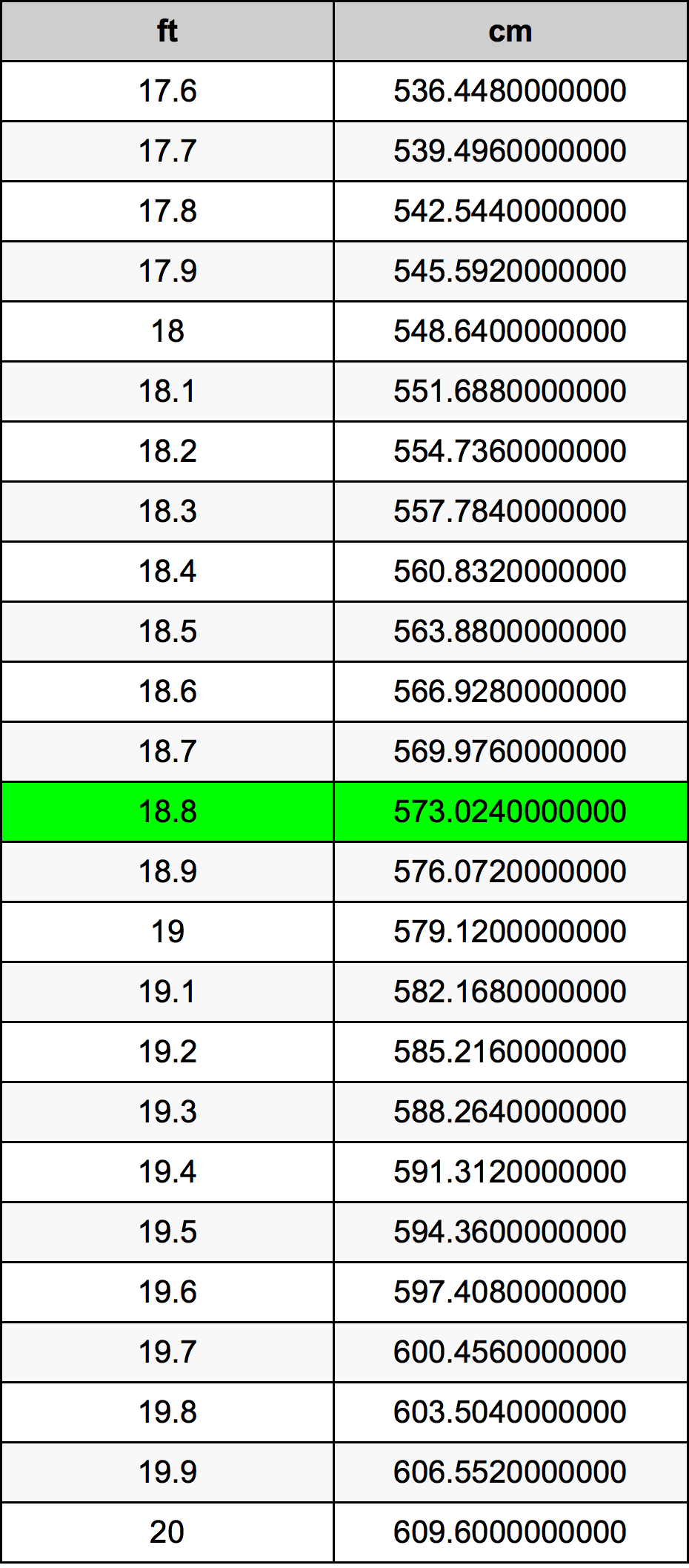Feet To Cm

# 18.8 ft to cm18.8 Feet to Centimeters

ft
=
cm

## How to convert 18.8 feet to centimeters?

 18.8 ft * 30.48 cm = 573.024 cm 1 ft
A common question is How many foot in 18.8 centimeter? And the answer is 0.6167979003 ft in 18.8 cm. Likewise the question how many centimeter in 18.8 foot has the answer of 573.024 cm in 18.8 ft.

## How much are 18.8 feet in centimeters?

18.8 feet equal 573.024 centimeters (18.8ft = 573.024cm). Converting 18.8 ft to cm is easy. Simply use our calculator above, or apply the formula to change the length 18.8 ft to cm.

## Convert 18.8 ft to common lengths

UnitUnit of length
Nanometer5730240000.0 nm
Micrometer5730240.0 µm
Millimeter5730.24 mm
Centimeter573.024 cm
Inch225.6 in
Foot18.8 ft
Yard6.2666666667 yd
Meter5.73024 m
Kilometer0.00573024 km
Mile0.0035606061 mi
Nautical mile0.0030940821 nmi

## What is 18.8 feet in cm?

To convert 18.8 ft to cm multiply the length in feet by 30.48. The 18.8 ft in cm formula is [cm] = 18.8 * 30.48. Thus, for 18.8 feet in centimeter we get 573.024 cm.

## 18.8 Foot Conversion Table## Alternative spelling

18.8 Foot to Centimeters, 18.8 Foot in Centimeters, 18.8 Feet to Centimeters, 18.8 Feet in Centimeters, 18.8 Foot to Centimeter, 18.8 Foot in Centimeter, 18.8 ft to Centimeters, 18.8 ft in Centimeters, 18.8 ft to Centimeter, 18.8 ft in Centimeter, 18.8 Feet to Centimeter, 18.8 Feet in Centimeter, 18.8 ft to cm, 18.8 ft in cm